# Introduction to HMMs in Bioinformatics

Feb. 12, 2013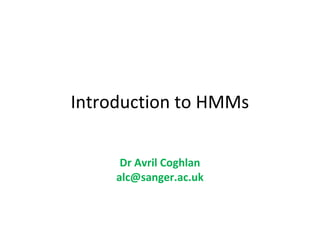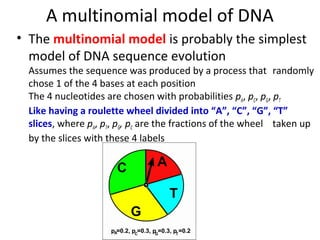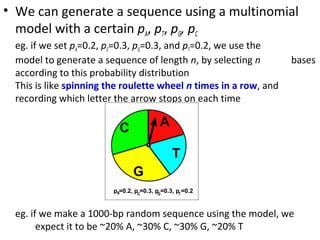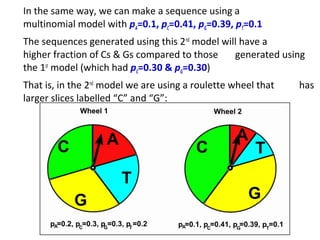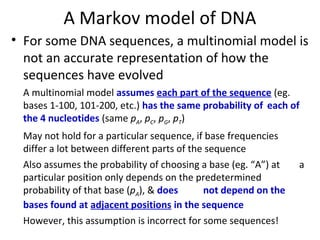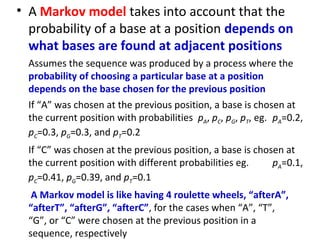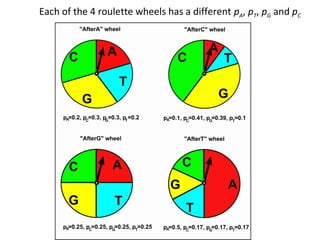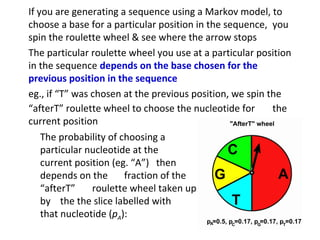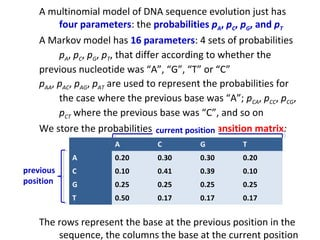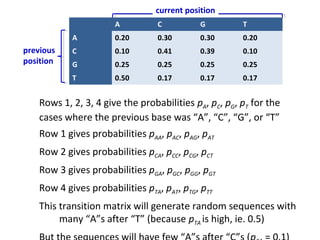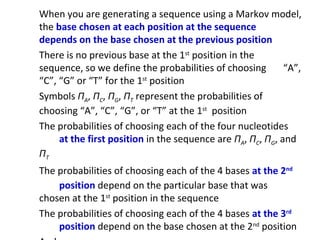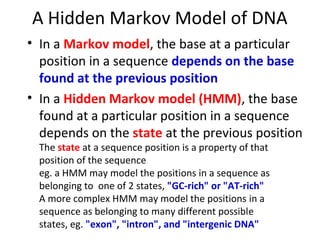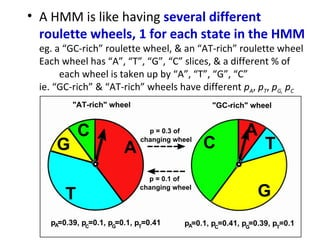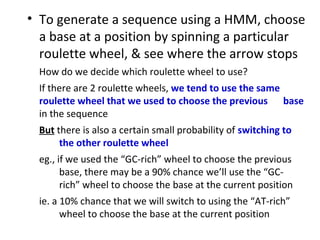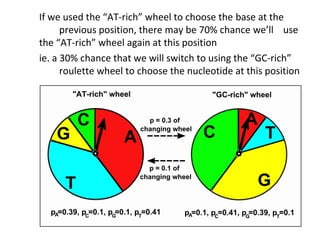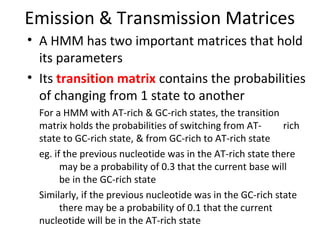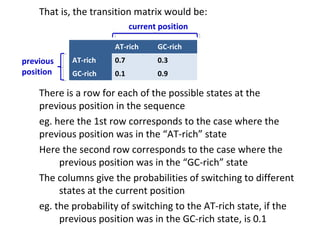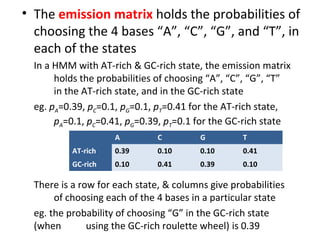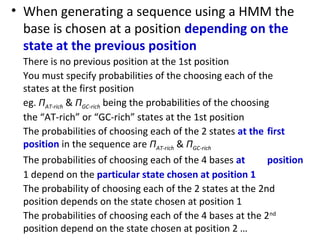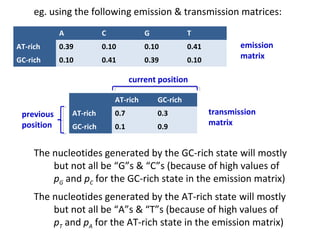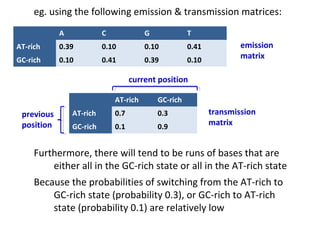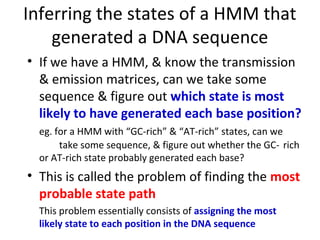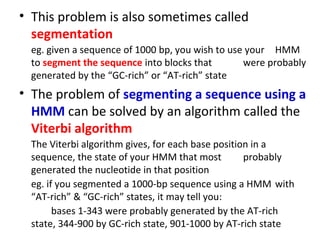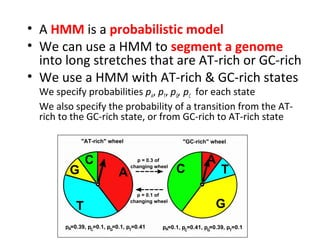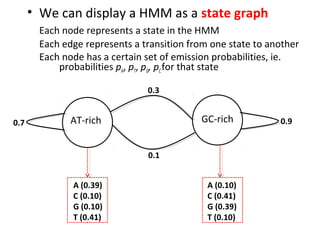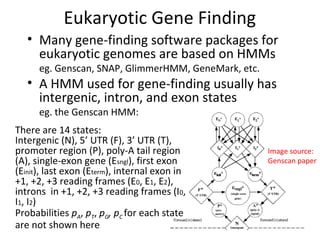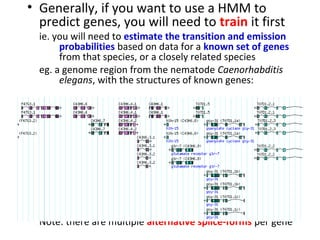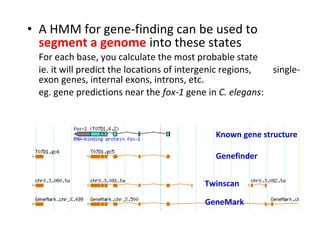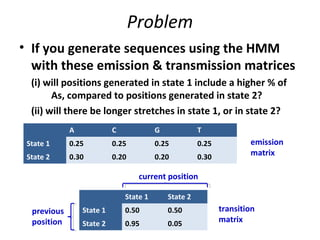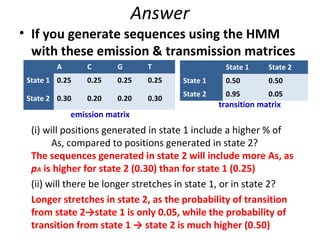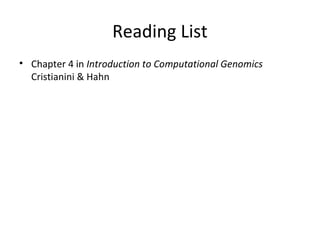1 of 31

### Introduction to HMMs in Bioinformatics

1. Introduction to HMMs Dr Avril Coghlan alc@sanger.ac.uk Note: this talk contains animations which can only be seen by downloading and using ‘View Slide show’ in Powerpoint
2. A multinomial model of DNA • The multinomial model is probably the simplest model of DNA sequence evolution Assumes the sequence was produced by a process that randomly chose 1 of the 4 bases at each position The 4 nucleotides are chosen with probabilities pA, pC, pG, pT Like having a roulette wheel divided into “A”, “C”, “G”, “T” slices, where pA, pT, pG, pC are the fractions of the wheel taken up by the slices with these 4 labels
3. • We can generate a sequence using a multinomial model with a certain pA, pT, pG, pC eg. if we set pA=0.2, pC=0.3, pG=0.3, and pT=0.2, we use the model to generate a sequence of length n, by selecting n bases according to this probability distribution This is like spinning the roulette wheel n times in a row, and recording which letter the arrow stops on each time eg. if we make a 1000-bp random sequence using the model, we expect it to be ~20% A, ~30% C, ~30% G, ~20% T
4. In the same way, we can make a sequence using a multinomial model with pA=0.1, pC=0.41, pG=0.39, pT=0.1 The sequences generated using this 2nd model will have a higher fraction of Cs & Gs compared to those generated using the 1st model (which had pC=0.30 & pG=0.30) That is, in the 2nd model we are using a roulette wheel that has larger slices labelled “C” and “G”:
5. A Markov model of DNA • For some DNA sequences, a multinomial model is not an accurate representation of how the sequences have evolved A multinomial model assumes each part of the sequence (eg. bases 1-100, 101-200, etc.) has the same probability of each of the 4 nucleotides (same pA, pC, pG, pT) May not hold for a particular sequence, if base frequencies differ a lot between different parts of the sequence Also assumes the probability of choosing a base (eg. “A”) at a particular position only depends on the predetermined probability of that base (pA), & does not depend on the bases found at adjacent positions in the sequence However, this assumption is incorrect for some sequences!
6. • A Markov model takes into account that the probability of a base at a position depends on what bases are found at adjacent positions Assumes the sequence was produced by a process where the probability of choosing a particular base at a position depends on the base chosen for the previous position If “A” was chosen at the previous position, a base is chosen at the current position with probabilities pA, pC, pG, pT, eg. pA=0.2, pC=0.3, pG=0.3, and pT=0.2 If “C” was chosen at the previous position, a base is chosen at the current position with different probabilities eg. pA=0.1, pC=0.41, pG=0.39, and pT=0.1 A Markov model is like having 4 roulette wheels, “afterA”, “afterT”, “afterG”, “afterC”, for the cases when “A”, “T”, “G”, or “C” were chosen at the previous position in a sequence, respectively
7. Each of the 4 roulette wheels has a different pA, pT, pG and pC
8. If you are generating a sequence using a Markov model, to choose a base for a particular position in the sequence, you spin the roulette wheel & see where the arrow stops The particular roulette wheel you use at a particular position in the sequence depends on the base chosen for the previous position in the sequence eg., if “T” was chosen at the previous position, we spin the “afterT” roulette wheel to choose the nucleotide for the current position The probability of choosing a particular nucleotide at the current position (eg. “A”) then depends on the fraction of the “afterT” roulette wheel taken up by the the slice labelled with that nucleotide (pA):
9. A multinomial model of DNA sequence evolution just has four parameters: the probabilities pA, pC, pG, and pT A Markov model has 16 parameters: 4 sets of probabilities pA, pC, pG, pT, that differ according to whether the previous nucleotide was “A”, “G”, “T” or “C” pAA, pAC, pAG, pAT are used to represent the probabilities for the case where the previous base was “A”; pCA, pCC, pCG, pCT where the previous base was “C”, and so on We store the probabilities in a Markov transition matrix: current position A C G T A 0.20 0.30 0.30 0.20 previous C 0.10 0.41 0.39 0.10 position G 0.25 0.25 0.25 0.25 T 0.50 0.17 0.17 0.17 The rows represent the base at the previous position in the sequence, the columns the base at the current position
10. current position A C G T A 0.20 0.30 0.30 0.20 previous C 0.10 0.41 0.39 0.10 position G 0.25 0.25 0.25 0.25 T 0.50 0.17 0.17 0.17 Rows 1, 2, 3, 4 give the probabilities pA, pC, pG, pT for the cases where the previous base was “A”, “C”, “G”, or “T” Row 1 gives probabilities pAA, pAC, pAG, pAT Row 2 gives probabilities pCA, pCC, pCG, pCT Row 3 gives probabilities pGA, pGC, pGG, pGT Row 4 gives probabilities pTA, pAT, pTG, pTT This transition matrix will generate random sequences with many “A”s after “T” (because pTA is high, ie. 0.5)
11. When you are generating a sequence using a Markov model, the base chosen at each position at the sequence depends on the base chosen at the previous position There is no previous base at the 1st position in the sequence, so we define the probabilities of choosing “A”, “C”, “G” or “T” for the 1st position Symbols ΠA, ΠC, ΠG, ΠT represent the probabilities of choosing “A”, “C”, “G”, or “T” at the 1st position The probabilities of choosing each of the four nucleotides at the first position in the sequence are ΠA, ΠC, ΠG, and ΠT The probabilities of choosing each of the 4 bases at the 2nd position depend on the particular base that was chosen at the 1st position in the sequence The probabilities of choosing each of the 4 bases at the 3rd position depend on the base chosen at the 2nd position
12. A Hidden Markov Model of DNA • In a Markov model, the base at a particular position in a sequence depends on the base found at the previous position • In a Hidden Markov model (HMM), the base found at a particular position in a sequence depends on the state at the previous position The state at a sequence position is a property of that position of the sequence eg. a HMM may model the positions in a sequence as belonging to one of 2 states, "GC-rich" or "AT-rich" A more complex HMM may model the positions in a sequence as belonging to many different possible states, eg. "exon", "intron", and "intergenic DNA"
13. • A HMM is like having several different roulette wheels, 1 for each state in the HMM eg. a “GC-rich” roulette wheel, & an “AT-rich” roulette wheel Each wheel has “A”, “T”, “G”, “C” slices, & a different % of each wheel is taken up by “A”, “T”, “G”, “C” ie. “GC-rich” & “AT-rich” wheels have different pA, pT, pG, pC
14. • To generate a sequence using a HMM, choose a base at a position by spinning a particular roulette wheel, & see where the arrow stops How do we decide which roulette wheel to use? If there are 2 roulette wheels, we tend to use the same roulette wheel that we used to choose the previous base in the sequence But there is also a certain small probability of switching to the other roulette wheel eg., if we used the “GC-rich” wheel to choose the previous base, there may be a 90% chance we’ll use the “GC- rich” wheel to choose the base at the current position ie. a 10% chance that we will switch to using the “AT-rich” wheel to choose the base at the current position
15. If we used the “AT-rich” wheel to choose the base at the previous position, there may be 70% chance we’ll use the “AT-rich” wheel again at this position ie. a 30% chance that we will switch to using the “GC-rich” roulette wheel to choose the nucleotide at this position
16. Emission & Transmission Matrices • A HMM has two important matrices that hold its parameters • Its transition matrix contains the probabilities of changing from 1 state to another For a HMM with AT-rich & GC-rich states, the transition matrix holds the probabilities of switching from AT- rich state to GC-rich state, & from GC-rich to AT-rich state eg. if the previous nucleotide was in the AT-rich state there may be a probability of 0.3 that the current base will be in the GC-rich state Similarly, if the previous nucleotide was in the GC-rich state there may be a probability of 0.1 that the current nucleotide will be in the AT-rich state
17. That is, the transition matrix would be: current position AT-rich GC-rich previous AT-rich 0.7 0.3 position GC-rich 0.1 0.9 There is a row for each of the possible states at the previous position in the sequence eg. here the 1st row corresponds to the case where the previous position was in the “AT-rich” state Here the second row corresponds to the case where the previous position was in the “GC-rich” state The columns give the probabilities of switching to different states at the current position eg. the probability of switching to the AT-rich state, if the previous position was in the GC-rich state, is 0.1
18. • The emission matrix holds the probabilities of choosing the 4 bases “A”, “C”, “G”, and “T”, in each of the states In a HMM with AT-rich & GC-rich state, the emission matrix holds the probabilities of choosing “A”, “C”, “G”, “T” in the AT-rich state, and in the GC-rich state eg. pA=0.39, pC=0.1, pG=0.1, pT=0.41 for the AT-rich state, pA=0.1, pC=0.41, pG=0.39, pT=0.1 for the GC-rich state A C G T AT-rich 0.39 0.10 0.10 0.41 GC-rich 0.10 0.41 0.39 0.10 There is a row for each state, & columns give probabilities of choosing each of the 4 bases in a particular state eg. the probability of choosing “G” in the GC-rich state (when using the GC-rich roulette wheel) is 0.39
19. • When generating a sequence using a HMM the base is chosen at a position depending on the state at the previous position There is no previous position at the 1st position You must specify probabilities of the choosing each of the states at the first position eg. ΠAT-rich & ΠGC-rich being the probabilities of the choosing the “AT-rich” or “GC-rich” states at the 1st position The probabilities of choosing each of the 2 states at the first position in the sequence are ΠAT-rich & ΠGC-rich The probabilities of choosing each of the 4 bases at position 1 depend on the particular state chosen at position 1 The probability of choosing each of the 2 states at the 2nd position depends on the state chosen at position 1 The probabilities of choosing each of the 4 bases at the 2nd position depend on the state chosen at position 2 …
20. eg. using the following emission & transmission matrices: A C G T AT-rich 0.39 0.10 0.10 0.41 emission GC-rich 0.10 0.41 0.39 0.10 matrix current position AT-rich GC-rich previous AT-rich 0.7 0.3 transmission position GC-rich 0.1 0.9 matrix The nucleotides generated by the GC-rich state will mostly but not all be “G”s & “C”s (because of high values of pG and pC for the GC-rich state in the emission matrix) The nucleotides generated by the AT-rich state will mostly but not all be “A”s & “T”s (because of high values of pT and pA for the AT-rich state in the emission matrix)
21. eg. using the following emission & transmission matrices: A C G T AT-rich 0.39 0.10 0.10 0.41 emission GC-rich 0.10 0.41 0.39 0.10 matrix current position AT-rich GC-rich previous AT-rich 0.7 0.3 transmission position GC-rich 0.1 0.9 matrix Furthermore, there will tend to be runs of bases that are either all in the GC-rich state or all in the AT-rich state Because the probabilities of switching from the AT-rich to GC-rich state (probability 0.3), or GC-rich to AT-rich state (probability 0.1) are relatively low
22. Inferring the states of a HMM that generated a DNA sequence • If we have a HMM, & know the transmission & emission matrices, can we take some sequence & figure out which state is most likely to have generated each base position? eg. for a HMM with “GC-rich” & “AT-rich” states, can we take some sequence, & figure out whether the GC- rich or AT-rich state probably generated each base? • This is called the problem of finding the most probable state path This problem essentially consists of assigning the most likely state to each position in the DNA sequence
23. • This problem is also sometimes called segmentation eg. given a sequence of 1000 bp, you wish to use your HMM to segment the sequence into blocks that were probably generated by the “GC-rich” or “AT-rich” state • The problem of segmenting a sequence using a HMM can be solved by an algorithm called the Viterbi algorithm The Viterbi algorithm gives, for each base position in a sequence, the state of your HMM that most probably generated the nucleotide in that position eg. if you segmented a 1000-bp sequence using a HMM with “AT-rich” & “GC-rich” states, it may tell you: bases 1-343 were probably generated by the AT-rich state, 344-900 by GC-rich state, 901-1000 by AT-rich state
24. • A HMM is a probabilistic model • We can use a HMM to segment a genome into long stretches that are AT-rich or GC-rich • We use a HMM with AT-rich & GC-rich states We specify probabilities pA, pT, pG, pC for each state We also specify the probability of a transition from the AT- rich to the GC-rich state, or from GC-rich to AT-rich state
25. • We can display a HMM as a state graph Each node represents a state in the HMM Each edge represents a transition from one state to another Each node has a certain set of emission probabilities, ie. probabilities pA, pT, pG, pC for that state 0.3 0.7 AT-rich GC-rich 0.9 0.1 A (0.39) A (0.10) C (0.10) C (0.41) G (0.10) G (0.39) T (0.41) T (0.10)
26. Eukaryotic Gene Finding • Many gene-finding software packages for eukaryotic genomes are based on HMMs eg. Genscan, SNAP, GlimmerHMM, GeneMark, etc. • A HMM used for gene-finding usually has intergenic, intron, and exon states eg. the Genscan HMM: There are 14 states: Intergenic (N), 5’ UTR (F), 3’ UTR (T), promoter region (P), poly-A tail region Image source: (A), single-exon gene (Esngl), first exon Genscan paper (Einit), last exon (Eterm), internal exon in +1, +2, +3 reading frames (E0, E1, E2), introns in +1, +2, +3 reading frames (I0, I1, I2) Probabilities pA, pT, pG, pC for each state are not shown here
27. • Generally, if you want to use a HMM to predict genes, you will need to train it first ie. you will need to estimate the transition and emission probabilities based on data for a known set of genes from that species, or a closely related species eg. a genome region from the nematode Caenorhabditis elegans, with the structures of known genes: Note: there are multiple alternative splice-forms per gene
28. • A HMM for gene-finding can be used to segment a genome into these states For each base, you calculate the most probable state ie. it will predict the locations of intergenic regions, single- exon genes, internal exons, introns, etc. eg. gene predictions near the fox-1 gene in C. elegans: Known gene structure Genefinder Twinscan GeneMark
29. Problem • If you generate sequences using the HMM with these emission & transmission matrices (i) will positions generated in state 1 include a higher % of As, compared to positions generated in state 2? (ii) will there be longer stretches in state 1, or in state 2? A C G T State 1 0.25 0.25 0.25 0.25 emission State 2 0.30 0.20 0.20 0.30 matrix current position State 1 State 2 previous State 1 0.50 0.50 transition position State 2 0.95 0.05 matrix
30. Answer • If you generate sequences using the HMM with these emission & transmission matrices A C G T State 1 State 2 State 1 0.25 0.25 0.25 0.25 State 1 0.50 0.50 State 2 0.95 0.05 State 2 0.30 0.20 0.20 0.30 transition matrix emission matrix (i) will positions generated in state 1 include a higher % of As, compared to positions generated in state 2? The sequences generated in state 2 will include more As, as pA is higher for state 2 (0.30) than for state 1 (0.25) (ii) will there be longer stretches in state 1, or in state 2? Longer stretches in state 2, as the probability of transition from state 2→state 1 is only 0.05, while the probability of transition from state 1 → state 2 is much higher (0.50)
31. Reading List • Chapter 4 in Introduction to Computational Genomics Cristianini & Hahn • Practical on HMMs in R in the Little Book of R for Bioinformatics: https://a-little-book-of-r-for- bioinformatics.readthedocs.org/en/latest/src/chapter10.html

### Editor's Notes

1. Genscan paper: http://www.bx.psu.edu/old/courses/bx-fall08/genscan.pdf